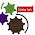## Tuesday, 23 August 2016

### Fibonacci Series Using Array And For Loop - C Programming Examples

As we have started C Programming coding with Example Series, We have published last posts

In this post we are doing again Fibonacci Series but with different approach.In last post "Fibonacci Progression Using While Loop" you will see how to generate Fibonacci Numbers by using concepts of  Do-While Loop.In this post we will talk about another loop i.e. For Loop and side by side Concept of Arrays will be discussed and demonstrated.

Fibonacci Series - Sequence ( Using Array And For Loop ) Video Tutorial(See C- Codes Below ):

For Loop Concept: FOR Loops are the most useful type. The syntax for a for loop is
for ( variable initialisation; condition; variable update ) {
Code to execute while the condition is true
}

A working Example of FOR Loop
Example:

#include <stdio.h>

int main()
{
int x;
/* The loop goes while x < 10, and x increases by one every loop*/
for ( x = 0; x < 10; x++ )
{
/* Keep in mind that the loop condition checks
the conditional statement before it loops again.*/
printf( "%d\n", x );
}
getchar();
}

Array Concept: Arrays may be defined as a kind of data structure that can store a fixed-size sequential collection of elements of the same type.An array is used to store a collection of data, but it is often more useful to think of an array as a collection of variables of the same type.

Arrays are of two types:
A. One-dimensional arrays
B. Multidimensional arrays

To declare an array in C, specifies the type of the elements and the number of elements required by an array as follows −

type arrayName [ arraySize ]

Initialising array in C using a single statement −
double MoneyBalance = {1000.0, 2.0, 3.4, 7.0, 50.0};

If you omit the size of the array, an array just big enough to hold the initialisation is created −

double MoneyBalance[] = {1000.0, 2.0, 3.4, 7.0, 50.0};
More of Fibonacci Sequence Tutorials.

Write A Program In C To Find Fibonacci Numbers Progression - Series - Sequence to Nth Term:C Codes are written and tested in TuboC/C++ IDE as you can see in Video:

C Program -

#include<stdio.h>
int main()
{
int i,range;
long int arr;

printf("\nEnter the number range: ");
scanf("%d",&range);

arr=0;
arr=1;

for(i=2;i<range;i++){
arr[i] = arr[i-1] + arr[i-2];
}

printf("Fibonacci series is: ");
for(i=0;i<range;i++)
printf("%ld ",arr[i]);
printf("\nFibonacci Ends \n\n");
delay(5000);
return 0;
}

Write A Program in C to Find Fibonacci series up to Nth terms (Use Of Array and FOR Loop)

Result:-

Enter the number range: 20
Fibonacci series is: 0 1 1 2 3 5 8 13 21 34 55 89 144 233 377 610 987 1597 2584 4181

1.That is an extremely smart written article. I will be sure to bookmark it and return to learn extra of your useful information. Thank you for the post. I will certainly return.

1.2.